"Double, or dual, number lines are an ideal "visual model" for solving mathematical problems involving equivalences, ratios, proportions, and more. Though double number lines are not mentioned specifically in the CCSS until Grade 6 (6.RP.3), they are a viable tool for mathematical understanding beginning as early as first grade.

One nifty innovation is the Base Ten Dual Number Line, a physical manipulative that allows students to place unit blocks and ten rods in upper and lower channels as they work out problems involving addition, subtraction, place value, and more.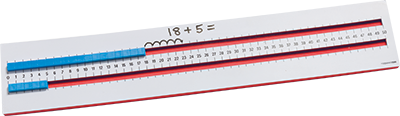Double number lines are ideal for demonstrating fraction equivalence (Grade 3) and equivalence between fractions, decimals, and percents (Grade 4). They are also ideal for solving problems involving conversion of measurements from a larger unit to a smaller unit and vice versa (Grade 4).

In Grade 6, students "connect their understanding of multiplication and division with ratio and rates" (CCSS-M, p. 39). Double number lines facilitate this work, setting the stage for Grade 7, in which students learn to represent proportional relationships by equations and graph these equations on the coordinate plane.

Sample Double Number Line Activities

Following are some sample problems that can be solved easily using double number lines.

Problem: I have three donuts and I am going to buy 12 more. How many donuts will I have?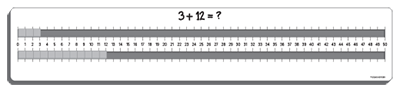Using the Base Ten Dual Number LIne, have the students illustrate the problem by showing the number line with 3 units in the top channel and one rod and 2 units in the bottom. They can then move the cubes from the bottom channel next to the cubes in the top channel to see that the total is 15. (1.OA.1)

## Inequalities

Problem: 34 > 28 Using the Base Ten Dual Number Line, ask the students to compare 34 and 28 using the blocks and two channels of the number line. They can then record their findings using the appropriate symbol (> or <). Repeat with other numbers below 50. (1.NBT.3)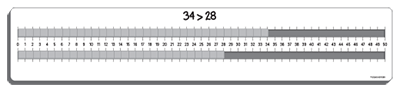## Fraction Equivalence

Problem: Is 2/6 equivalent to 1/3? Prove it by drawing a double number line diagram. (3.NF.3.a)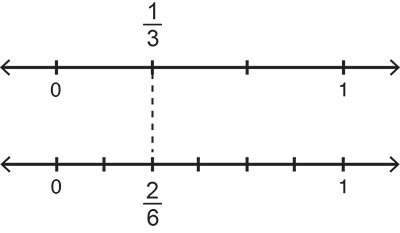Similarly, in Grade 4, double--or triple--number line diagrams can be employed to show equivalence between fractions, decimals, and percentages. (4.NF.5)

## Ratio

Problem: If you are using 1 cup of orange juice to 3 cups of grape juice, how many cups of grape juice will you need if you have 4 cups of orange juice? (6.RP.3.b)

Using additive reasoning, the solution to your problem would look like this on a double number line diagram: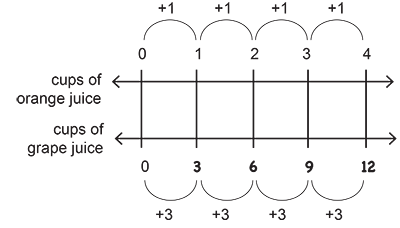## Unit Rate

Problem: A plane flew 800 miles in 2 hours. If it continues to fly at the same rate of speed, how long will it take for the plane to fly 3200 miles? (6.RP.3.b)

Using multiplicative reasoning, the solution to your problem would look like this on a double number line diagram: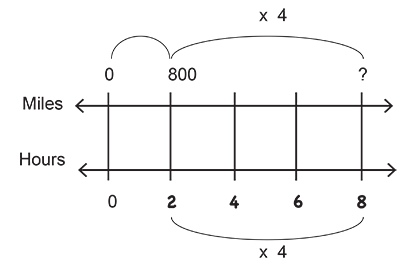## Summary

As visual models, double number lines have many applications across Grades 1 through 6. The work accomplished using double number lines helps prepare students for the more abstract algebraic work with equations in Grades 7 and beyond."